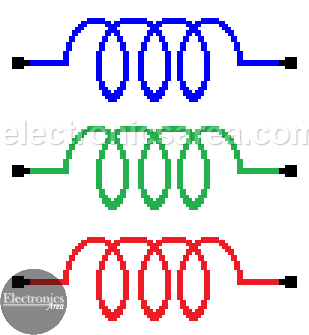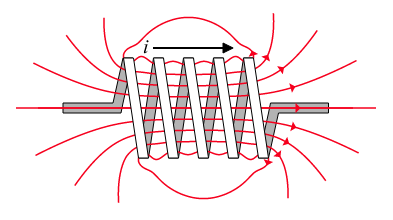# What is an Inductor – Coil?

The capacitor storages energy in the form of electric field, however, inductor – coil stores energy in form of magnetic field due to the self-induction phenomenon. A cable where a current circulates, has on its surroundings a magnetic field. The direction of flow of the   magnetic field is establishes by the Right hand law.

Since the inductor is made of cable spires, the magnetic field circulates throughout the center of the coil and it runs all the wayout surrounding the spires and going back to the center.

One of the interesting features is its opposition to abrupt changes in the electric current flowing through them. This means that when we try to modify the current that circulates through them (for example: to be connected and disconnected to a power source of continuous current), it will try to maintain its previous condition.

This event occurs on an ongoing basis, when the inductor is connected to a source of alternating current and it causes a phase difference between the voltage applied to it and the current flowing through.

In other words: Inductor is an element that reacts against the changes of the electric current that flows through it, creating a voltage on its terminals that opposes the applied voltage. This is proportional to the rate of change of the current.The inductor is a passive electric component made of a conductive wire
wrapped into a spring like form.

## What is inductance? – Inductance formula

Inductance is the property of an electrical conductor by which a change in current through it induces an electromotive force (EMF) in both the conductor itself and in any nearby conductors by mutual inductance.

An inductor has an inductance, (L) of one Henry, (1H) when the current flowing through the coil changes at a rate of one ampere/second, (A/s). This change induces a voltage of one volt, (1V) in it. (VL).

VL = -L di/dt

By increasing the number turns within a coil, we can increase the coils inductance. The relationship between inductance (self inductance) and tne number of turns is:

Inductance formula: L = NΦ/I

where:

• L is the inductance in Henries
• N is the number of turns
• Φ is the magnetic flux in Webers (Wb)
• I is the current in Amperes

## Inductance Units

The inductance measures the value of opposition of the inductor to the flow of the electric current and it is measured in henry (H). We may find values of milihenrys (mH), microhenrys (uH). This value depends on the following:

• The number of turns that the inductor has (the more turns, the bigger the inductance).
• The diameter of the spires (turn). The more diameter, the bigger the inductance.
• The length of the cable that the coil is made of.
• The type of material that the nucleus is made of, if any.

## Magnetic field lines & Counter electromotive force (CEMF)

Lines of force of the magnetic field expands starting in the center of the electrical conductor and then moving away.

First the lines of force pass through the conductor itself and then through the air. While these lines of force are in the electrical conductor, an electromotive force (emf) is generated in the conductor itself. The voltage generated has an opposite direction of the electric current. Because of this, the force is called: Counter-electromotive force (CEMF).By inductiveload – Own drawing, done in Inkscape 0.44, Public Domain, Link

This effect causes that temporarily the maximum value of current in the conductor is not reached. When, eventually, the current variation disappears (the current has a constant value), the lines of force no longer expand and the counter electromotive force disappears.

When current begins to flow through the conductor, the lines of force of the magnetic field begin to expand rapidly and a large counter electromotive force is achieved. At this time the counter electromotive force is almost equal to the applied voltage source. Thus, the voltage source and the counter electromotive force almost cancel each other and the current flow is small.

After some time, the lines of magnetic field reaches its maximum value, the Counter electromotive force (CEMF) no longer is generated and the only electromotive force is the voltage source. At this time, the maximum current flows on the circuit because there is no opposition of the inductance.

Again, this property of opposing the current changes, self-inducing an electromotive force in the opposite direction (counter electromotive force) is called inductance. The unit of inductance is the henry (H) and is represented by the capital letter “L”.

## Inductance calculation example

Example

What is the self inductance of a 200 turn copper coil which produces a magnetic flux of 2 mWb when passing a DC current of 5 amps?

• N = 200 turns
• Φ = 2 mWb = 0,002 Webers
• I = 5 Amps

Using the Inductance formula:

L = NΦ/I = (200)(0.002)/5 = 0.08 Henries = 80 mH.

## EMF (VL) calculation example

Example # 1

What is the self-induced EMF produced in a 250mH inductor when passing a DC current of 10 amp after a time of 30 ms?

Using the above formula:  VL = – L di/dt,  where

• L = 250 mH
• di = 10 Amp
• dt = 30 ms

EMF = VL = – L di/dt = – (250 x 10-3 H) x (10 A) / (30 x 10-3 s) = – 83.33 Volts.

Example # 2

What is the self-induced EMF produced in a 200 mH coil when passing a DC current of 3 Amps after a time of 20 ms?

• L = 200 mH = 0.2 Henries
• I = 3 Amps
• t = 20 ms = 0.02 s

EMF = VL = – L di/dt = – (0.2)(3/0.02) = – 30 Volts.

inductor-and-inductance

inductor-coil-inductance

•
•
•
•
•
•
•
•
•
•
•
•
•
•
•
•
•
•